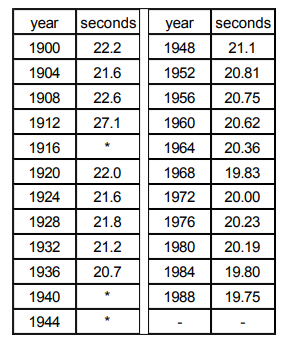# IMAT 2013 Q15 [Olympic Games]

The table below shows the winning time for the men’s 200m run in the Olympic Games since 1900, when the event was first held, until 1988.(* Olympics not held in these years.)

What is the longest number of years for which the Olympic record stood unbroken?

A. 6
B. 16
C. 24
D. 28
E. 20

Simple steps to solve word problems:

• Underline key information
• Determine what they are trying to ask, and what you will need to solve it
• Eliminate any non-essential information
• Draw a picture, graph, or equation
• In moments of high stress like exam taking, always work with the paper they give you to avoid careless mistakes.
• Solve.

Approach: count the interval between every record, and compare at the end. Also note that when you tie a record, you are not breaking it.

21.6 s

From 19041932 = 28 years

Right away we know that 28 years is the answer because teh question is asking for the longest interval, and out of our options, 28 is the longest. For completeness, here is the rest of the solution:

20.7 s

From 19361960 = 24 years

20.62 s

From 19601964 = 4 years

20.36 s

From 19641968 = 4 years

19.83 s

From 1968 → \$1988 = 20 years

\fcolorbox{red}{grey!30}{Therefore D, \$28\$ is correct.}